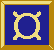# ¤ Fudget Library 0.18.4 Reference Manual ¤

Created from the Fudget Library sources on 2023-06-21 17:48

## GuiElems

Buttons:
`quitButtonF :: F Click b`
Selecting from dynamic lists of alternatives:
`oldFilePickF :: F FilePath (InputMsg String)`
Miscellaneous (the rest):
```oldButtonF :: (Graphic e, FontGen p1, Show p1, Show p2, ColorGen p2) =>
Alignment ->
Distance ->
p1 -> ColorSpec -> p2 -> [(ModState, KeySym)] -> e -> F e Click
oldPopupMenuF :: (Eq b1, Graphic b2, Foldable t) =>
ColorName ->
Bool ->
FontName ->
Button ->
ModState ->
t (ModState, KeySym) ->
[(b1, b3)] -> (b1 -> b2) -> F c d -> F ([(b1, b4)] ⊕ c) (b1 ⊕ d)```

## Combinators

Miscellaneous (the rest):
```inputListLF :: Eq a => Placer -> [(a, InF b c)] -> F [(a, b)] (InputMsg [(a, c)])
inputPairLF :: Orientation -> InF a1 b1 -> InF a2 b2 -> F (a1, a2) (InputMsg (b1, b2))```

## InfixOps

Miscellaneous (the rest):
```>#+< :: (F a b, Orientation) -> F c d -> F (a ⊕ c) (b ⊕ d)
>#==< :: (F d f, Orientation) -> F e d -> F e f```

## Layout

Miscellaneous (the rest):
```data Orientation = ...
compLF :: (F a b, Orientation) -> F c d -> F (a ⊕ c) (b ⊕ d)
listLF :: Eq a => Placer -> [(a, F b c)] -> F (a, b) (a, c)
serCompLF :: (F d f, Orientation) -> F e d -> F e f
untaggedListLF :: Placer -> [F a b] -> F (Int, a) b```

## Containers

Miscellaneous (the rest):
```oldHscrollF :: Bool -> (Point, Point) -> F b d -> F b d
oldScrollF :: Bool -> (Point, Point) -> F b d -> F b d
oldVscrollF :: Bool -> (Point, Point) -> F b d -> F b d
scrollShellF :: String -> (Point, Point) -> F c d -> F c d```

## StreamProc

Stream processor equivalents of some common list processing functions:
```concSP :: SP [b] b
concmapSP :: (t -> [b]) -> SP t b
mapstateSP :: (t -> a -> (t, [b])) -> t -> SP a b```